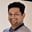Related Tags

java
communitycreator
binary search tree

# How to check if a binary tree is a binary search tree in JavaTarun Telang

Before we get into the details of the algorithm, let’s first look into some of the basics related to the tree data structure.

## Tree

A tree is a recursive data structure used to represent a hierarchy of data. It is made of a collection of nodes linked together by edges to represent a hierarchy with no cycles. Each node may contain some data or references to other nodes.

Root node – A node with no parent.

Parent node – A node that references some other node is the referenced node’s parent.

Children nodes – A node that was referenced by another node.

Sibling nodes – Nodes that have the same parent.

Leaf nodes – A node that does not reference some other nodes. Leaf Nodes do not have any children.

## Binary tree

A tree whose every node has, at most, two children is known as a binary tree.

### Binary search tree

A binary search tree is a special kind of binary tree in which, for each node, the following properties must be satisfied:

1. The value of all the nodes in the left subtree should be less than that of the parent node.
2. The value of all the nodes in the right subtree should be greater than that of the parent node.
3. The left and right subtrees must also be binary search trees.

Note: Duplicate values are not allowed.

Properties of a binary search tree

The tree below is an example of a valid binary search tree:

Binary Search Tree

## Code

One of the most straightforward approaches used to verify a binary search tree is to make recursive calls to see if its node values are within a range of maximum and minimum values, as permitted by its definition.

To understand this better, take a look at the code example below:


class BinaryTree {
static class Node {
int value;
Node left;
Node right;
Node(int value) {
this.value = value;
}
}

public static boolean isValidBST(Node root) {
return isValidBST(root, null, null);
}

public static boolean isValidBST(Node root, Integer max, Integer min) {

// an empty binary trees is a valid BST.
if (root == null) return true;

if (max != null && root.value >= max) return false;

if (min != null && root.value <= min ) return false;

return isValidBST(root.left, root.value, min) &&
isValidBST(root.right, max, root.value);
}

//      10
//      / \
//     5  15
//    /\  / \
//   2  7 13 22
//  /      \
// 1       21
public static void main( String args[] ) {
// Creating a binary tree
Node root = new Node(10);
root.left = new Node(5);
root.right = new Node(15);
root.left.left = new Node(2);
root.left.left.left = new Node(1);
root.left.right = new Node(7);
root.right.left = new Node(13);
root.right.left.right = new Node(14);
root.right.right = new Node(21);

if (BinaryTree.isValidBST(root))
System.out.println("A valid BST");
else
System.out.println("Not a valid BST");
}
}
Code to verify a Binary Search Tree

## Time and space complexity

The above algorithm runs in linear time, i.e, $O(N)$ and has a linear space complexity, i.e., $O(N)$.

RELATED TAGS

java
communitycreator
binary search tree

CONTRIBUTORTarun Telang
RELATED COURSES

View all Courses

Keep Exploring

Learn in-demand tech skills in half the time